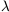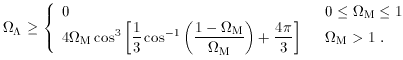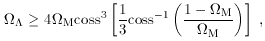2.2 Model universes and their fates

In the remainder of this section we will explore the behavior of universes dominated by matter and vacuum energy,=M += 1 -k. According to (32), a positive cosmological constant accelerates the universal expansion, while a negative cosmological constant and/or ordinary matter tend to decelerate it. The relative contributions of these components change with time; according to (28) we have(33)

For< 0, the universe will always recollapse to a Big Crunch, either because there is a sufficiently high matter density or due to the eventual domination of the negative cosmological constant. For> 0 the universe will expand forever unless there is sufficient matter to cause recollapse beforebecomes dynamically important. For= 0 we have the familiar situation in whichM1 universes expand forever andM > 1 universes recollapse; notice, however, that in the presence of a cosmological constant there is no necessary relationship between spatial curvature and the fate of the universe. (Furthermore, we cannot reliably determine that the universe will expand forever by any set of measurements ofandM; even if we seem to live in a parameter space that predicts eternal expansion, there is always the possibility of a future phase transition which could change the equation of state of one or more of the components.)

GivenM, the value offor which the universe will expand forever is given by(34)

Conversely, if the cosmological constant is sufficiently large compared to the matter density, the universe has always been accelerating, and rather than a Big Bang its early history consisted of a period of gradually slowing contraction to a minimum radius before beginning its current expansion. The criterion for there to have been no singularity in the past is(35)

where ``coss'' represents cosh whenM < 1/2, and cos whenM > 1/2.

The dynamics of universes with=M +are summarized in Figure (1), in which the arrows indicate the evolution of these parameters in an expanding universe. (In a contracting universe they would be reversed.)Figure 1. Dynamics for=M +. The arrows indicate the direction of evolution of the parameters in an expanding universe.

This is not a true phase-space plot, despite the superficial similarities. One important difference is that a universe passing through one point can pass through the same point again but moving backwards along its trajectory, by first going to infinity and then turning around (recollapse).

Figure (1) includes three fixed points, at (M,) equal to (0, 0), (0, 1), and (1, 0). The attractor among these at (1, 0) is known as de~Sitter space - a universe with no matter density, dominated by a cosmological constant, and with scale factor growing exponentially with time. The fact that this point is an attractor on the diagram is another way of understanding the cosmological constant problem. A universe with initial conditions located at a generic point on the diagram will, after several expansion times, flow to de Sitter space if it began above the recollapse line, and flow to infinity and back to recollapse if it began below that line. Since our universe has undergone a large number of e-folds of expansion since early times, it must have begun at a non-generic point in order not to have evolved either to de Sitter space or to a Big Crunch. The only other two fixed points on the diagram are the saddle point at (M,) = (0, 0), corresponding to an empty universe, and the repulsive fixed point at (M,) = (1, 0), known as the Einstein-de~Sitter solution. Since our universe is not empty, the favored solution from this combination of theoretical and empirical arguments is the Einstein-de~Sitter universe. The inflationary scenario [21, 22, 23] provides a mechanism whereby the universe can be driven to the lineM += 1 (spatial flatness), so Einstein-de~Sitter is a natural expectation if we imagine that some unknown mechanism sets= 0. As discussed below, the observationally favored universe is located on this line but away from the fixed points, near (M,) = (0.3, 0.7). It is fair to conclude that naturalness arguments have a somewhat spotty track record at predicting cosmological parameters.